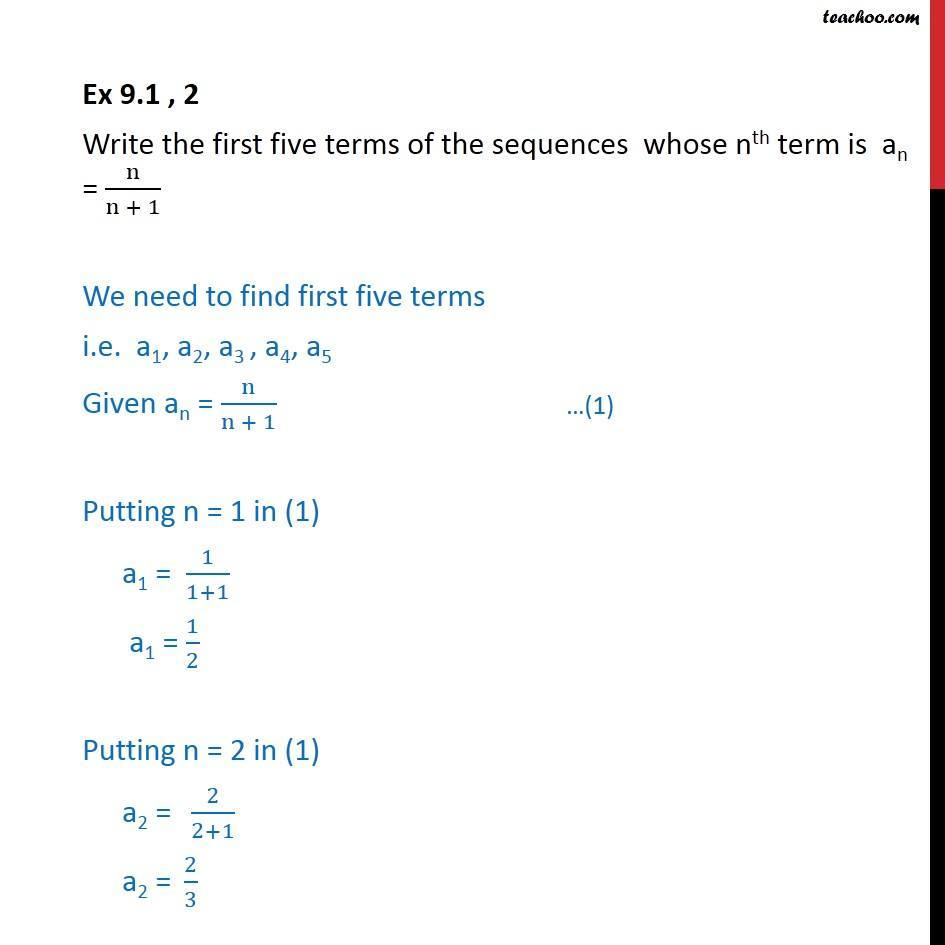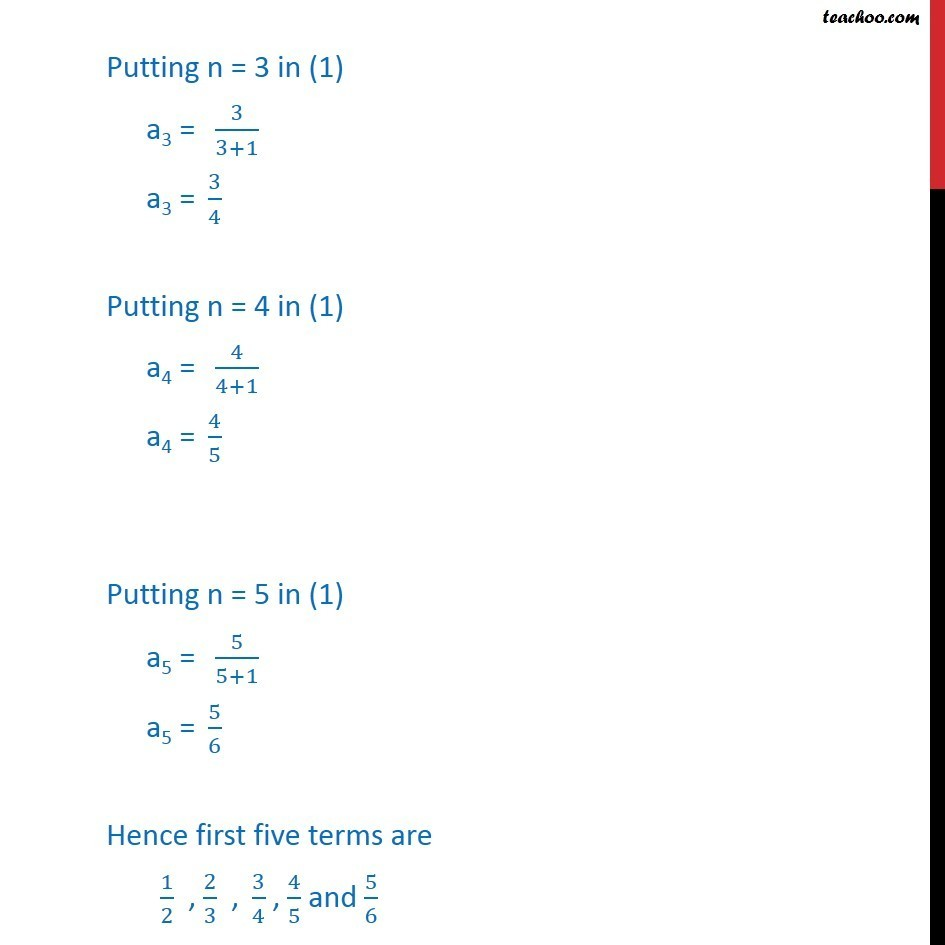Finding Sequences

Chapter 9 Class 11 Sequences and Series
Concept wiseIntroducing your new favourite teacher - Teachoo Black, at only ₹83 per month

### Transcript

Ex 9.1 , 2 Write the first five terms of the sequences whose nth term is an = n/(n + 1) We need to find first five terms i.e. a1, a2, a3 , a4, a5 Given an = n/(n + 1) Putting n = 1 in (1) a1 = 1/(1+1) a1 = 1/2 Putting n = 2 in (1) "a2 = " 2/(2+1) " " a2 "= " 2/3 Putting n = 3 in (1) "a3 = " 3/(3+1) " " a3 "= " 3/4 Putting n = 4 in (1) "a4 = " 4/(4+1) " " a4 "= " 4/5 Putting n = 5 in (1) "a5 = " 5/(5+1) a5 "= " 5/6 Hence first five terms are 1/2 , 2/3 ," " 3/4 , 4/5 and 5/6